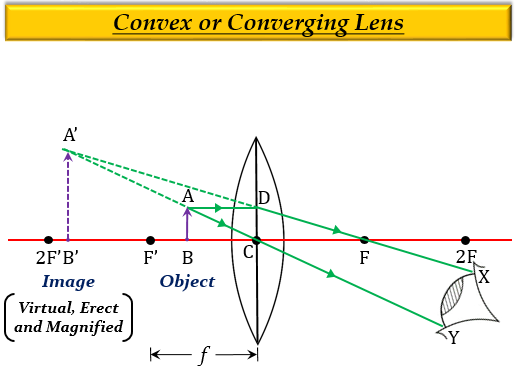# Describe with the help of a ray diagram the nature, size and position of the image formed when an object is placed in front of a convex lens between focus and optical centre. State three characteristics of the image formed.

The ray-diagram showing the nature, size, and position of the image formed when an object is placed in front of a convex lens between focus $F'$ and optical centre $C$.Characteristics of the image so formed are:

1. Nature of image $-$ virtual and erect.

2. Size of Image $-$ larger than the object (enlarged).

3. Position of Image $-$ Behind the object (on the left side).

Explanation

When an object is placed at a distance less than the focus i.e., between optical centre, $C$ and focus, $F'$ of a convex lens, then the image formed is virtual, erect, larger than the object (enlarged or magnified), and behind the object (on the left side of the lens).

Updated on: 10-Oct-2022

67 Views